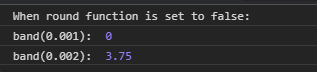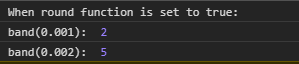Related Articles

# D3.js band.round() Function

• Last Updated : 24 Aug, 2020

The band.round() function is used to set the round true or false i.e it enables or disables the rounding. If the round is et to true then the start and stop of each band will be an integer.

Syntax:

`band.round([round]);`

Parameters: This function accepts single parameters as given above and described below:

• round: This parameter accepts a boolean value of true or false.

Return Values: This function does not return anything.

Below given are a few examples of the function given above.

Example 1:

 ` ``<``html` `lang` `= ``"en"``> ``<``head``> ``    ``<``meta` `charset` `= ``"UTF-8"` `/> ``    ``<``meta` `name` `= ``"viewport"``        ``path1tent = "``width``=``device``-width, ``        ``initial-scale` `= ``1``.0"/> ``    ``<``script` `src` `=``    ``"https://d3js.org/d3.v4.min.js"``>``    ````     ` ` ``<``body``> ``    ``<``script``> ``    ``// Creating the band scale with``    ``// specified domain and range.``        ``var band = d3.scaleBand()``          ``.domain([0.001, 0.002, 0.003, 0.004])``        ``// When range is in string``                    ``.range(["0", "15"]);``       ` `console.log("When round function is set to false:");``      ``// Setting round to false``        ``band.round(false);``       ` `        ``console.log("band(0.001): ", band(0.001));``        ``console.log("band(0.002): ", band(0.002));``    `` `` ```

Output:Example 2:

 ` ``<``html` `lang` `= ``"en"``> ``<``head``> ``    ``<``meta` `charset` `= ``"UTF-8"` `/> ``    ``<``meta` `name` `= ``"viewport"``        ``path1tent = "``width``=``device``-width, ``        ``initial-scale` `= ``1``.0"/> ``    ``<``script` `src` `=``    ``"https://d3js.org/d3.v4.min.js"``>``    ```` ``<``body``> ``    ``<``script``> ``    ``// Creating the band scale with ``   ``//specified domain and range.``        ``var band = d3.scaleBand()``   ``.domain([0.001, 0.002, 0.003, 0.004])``        ``// When range is in string``                    ``.range(["0", "15"]);``       ` ` ``console.log("When round function is set to true:");``      ``// Setting round to true``        ``band.round(true);``       ` `        ``console.log("band(0.001): ", band(0.001));``        ``console.log("band(0.002): ", band(0.002));``    `` `` ```

Output:Hey geek! The constant emerging technologies in the world of web development always keeps the excitement for this subject through the roof. But before you tackle the big projects, we suggest you start by learning the basics. Kickstart your web development journey by learning JS concepts with our JavaScript Course. Now at it’s lowest price ever!

My Personal Notes arrow_drop_up